JEE  >  JEE Main 2017 Question Paper with Solutions (9th April)

# JEE Main 2017 Question Paper with Solutions (9th April)

Test Description

## 90 Questions MCQ Test JEE Main & Advanced Mock Test Series | JEE Main 2017 Question Paper with Solutions (9th April)

JEE Main 2017 Question Paper with Solutions (9th April) for JEE 2023 is part of JEE Main & Advanced Mock Test Series preparation. The JEE Main 2017 Question Paper with Solutions (9th April) questions and answers have been prepared according to the JEE exam syllabus.The JEE Main 2017 Question Paper with Solutions (9th April) MCQs are made for JEE 2023 Exam. Find important definitions, questions, notes, meanings, examples, exercises, MCQs and online tests for JEE Main 2017 Question Paper with Solutions (9th April) below.
Solutions of JEE Main 2017 Question Paper with Solutions (9th April) questions in English are available as part of our JEE Main & Advanced Mock Test Series for JEE & JEE Main 2017 Question Paper with Solutions (9th April) solutions in Hindi for JEE Main & Advanced Mock Test Series course. Download more important topics, notes, lectures and mock test series for JEE Exam by signing up for free. Attempt JEE Main 2017 Question Paper with Solutions (9th April) | 90 questions in 180 minutes | Mock test for JEE preparation | Free important questions MCQ to study JEE Main & Advanced Mock Test Series for JEE Exam | Download free PDF with solutions
 1 Crore+ students have signed up on EduRev. Have you?
JEE Main 2017 Question Paper with Solutions (9th April) - Question 1

### A physical quantity P is described by the relation P  a1/2 b2 c3 d−4 If the relative errors in the measurement of a, b, c and d respectively, are 2%, 1%, 3% and 5%, then the relative error in P will be:

Detailed Solution for JEE Main 2017 Question Paper with Solutions (9th April) - Question 1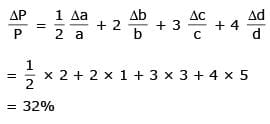JEE Main 2017 Question Paper with Solutions (9th April) - Question 2

### A car is standing 200 m behind a bus, which is also at rest. The two start moving at the same instant but with different forward accelerations. The bus has acceleration 2 m/s2 and the car has acceleration 4 m/s2. The car will catch up with the bus after a time of:

JEE Main 2017 Question Paper with Solutions (9th April) - Question 3

### Two particles A and B of equal mass M are moving with the same speed v as shown in the figure. They collide completely inelastically and move as a single particle C. The angle θ that the path of C makes with the X-axis is given by: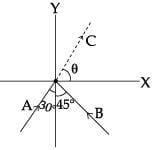JEE Main 2017 Question Paper with Solutions (9th April) - Question 4

The machine as shown has 2 rods of length 1 m connected by a pivot at the top. The end of one rod is connected to the floor by a stationary pivot and the end of the other rod has a roller that rolls along the floor in a slot. As the roller goes back and forth, a 2 kg weight moves up and down. If theroller is moving towards right at a constant speed, the weight moves up with a: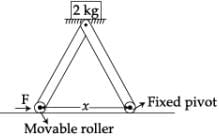JEE Main 2017 Question Paper with Solutions (9th April) - Question 5

A conical pendulum of length 1 m makes an angle θ=45o w.r.t. Z-axis and moves in a circle in the XY plane. The radius of the circle is 0.4 m and its center is vertically below O. The speed of the pendulum, in its circular path, will be: (Take g=10 ms−2)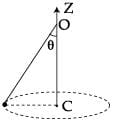JEE Main 2017 Question Paper with Solutions (9th April) - Question 6

A circular hole of radius R/4 is made in athin uniform disc having mass M and radius R, as shown in figure. The moment of inertia of the remaining portion of the disc about an axis passing through the point O and perpendicular to the plane of the disc is: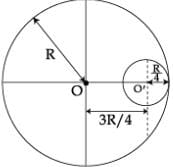JEE Main 2017 Question Paper with Solutions (9th April) - Question 7

The mass density of a spherical body is given by ρ (r) = k/r for r ≤ R and ρ (r)=0 for r > R, where r is the distance from the centre. The correct graph that describes qualitatively the acceleration, a, of a test particle as a function of r is:

JEE Main 2017 Question Paper with Solutions (9th April) - Question 8

A steel rail of length 5 m and area of cross-section 40cm2 is prevented from expanding along its length while the temperature rises by 10oC. If coefficient of linear expansion and Young’s modulus of steel are 1.2×10−5 K−1 and 2×1011 Nm−2 respectively, the force developed in the rail is approximately :

Detailed Solution for JEE Main 2017 Question Paper with Solutions (9th April) - Question 8

F = yA∝ Δt
= 2 × 1011 × 40 × 10-4 × 1.2 × 10-5 × 10
= 9.6 × 104 = 1 × 105 N

JEE Main 2017 Question Paper with Solutions (9th April) - Question 9

Two tubes of radii r1 and r2, and lengths l1 and l2, respectively, are connected in series and a liquid flows through each of them in stream line conditions. P1 and P2 are pressure differences across the two tubes.
If P2 is 4P1 and l2 is l1/4 , then the radius r2 will be equal to:

Detailed Solution for JEE Main 2017 Question Paper with Solutions (9th April) - Question 9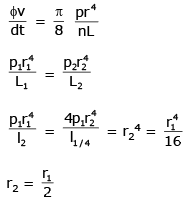JEE Main 2017 Question Paper with Solutions (9th April) - Question 10

For the P-V diagram given for an ideal gas,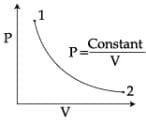out of the following which one correctly represents the T-P diagram?

JEE Main 2017 Question Paper with Solutions (9th April) - Question 11

N moles of a diatomic gas in a cylinder are at a temperature T. Heat is supplied to the cylinder such that the temperature remains constant but n moles of the diatomic gas get converted into monoatomic gas. What is the change in the total kinetic energy of the gas?

Detailed Solution for JEE Main 2017 Question Paper with Solutions (9th April) - Question 11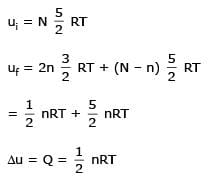JEE Main 2017 Question Paper with Solutions (9th April) - Question 12

A block of mass 0.1 kg is connected to an elastic spring of spring constant 640 Nm−1 and oscillates in a damping medium of damping constant 10−2 kg s−1.  The system dissipates its energy gradually.  The time taken for its mechanical energy of vibration to drop to half of its initial value, is closest to :

JEE Main 2017 Question Paper with Solutions (9th April) - Question 13

A standing wave is formed by the superposition of two waves travelling in opposite directions. The transverse displacement is given by y(x,t) = 0.5 sin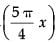cos(200 πt). What is the speed of the travelling wave moving in the positive x direction? (x and t are in meter and second, respectively.)

JEE Main 2017 Question Paper with Solutions (9th April) - Question 14

Four closed surfaces and corresponding charge distributions are shown below.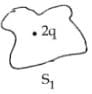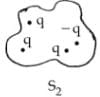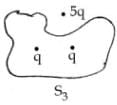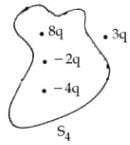Let the respective electric fluxes through the surfaces be Φ1, Φ2, Φ3 and Φ4. Then:

JEE Main 2017 Question Paper with Solutions (9th April) - Question 15

A combination of parallel plate capacitors is maintained at a certain potential difference.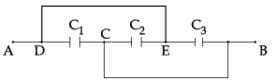When a 3 mm thick slab is introduced between all the plates, in order to maintain the same potential difference, the distance between the plates is increased by 2.4 mm. Find the dielectric constant of the slab.

JEE Main 2017 Question Paper with Solutions (9th April) - Question 16

A uniform wire of length l and radius r has a resistance of 100 Ω. It is recast into a wire of radius r/2. The resistance of new wire will be:

JEE Main 2017 Question Paper with Solutions (9th April) - Question 17

The figure shows three circuits I, II and III which are connected to a 3V battery.  If the powers dissipated by the configurations I, II and III are P1, P2 and P3 respectively, then: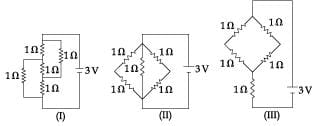JEE Main 2017 Question Paper with Solutions (9th April) - Question 18

A negative test charge is moving near a long straight wire carrying a current. The force acting on the test charge is parallel to the direction of the current. The motion of the charge is :

Detailed Solution for JEE Main 2017 Question Paper with Solutions (9th April) - Question 18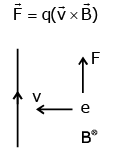JEE Main 2017 Question Paper with Solutions (9th April) - Question 19

A uniform magnetic field B of 0.3 T is along the positive Z-direction. A rectangular loop (abcd) of sides 10 cm×5 cm carries a current I of 12 A. Out of the following different orientations which one corresponds to stable equilibrium?

JEE Main 2017 Question Paper with Solutions (9th April) - Question 20

A sinusoidal voltage of peak value 283 V and angular frequency 320/s is applied to a series LCR circuit. Given that R=5 Ω, L=25 mH and C=1000 µF. The total impedance, and phase difference between the voltage across the source and the current will respectively be :

JEE Main 2017 Question Paper with Solutions (9th April) - Question 21

The electric field component of a monochromatic radiation is given by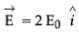cos kz cos ωt

JEE Main 2017 Question Paper with Solutions (9th April) - Question 22

In an experiment a convex lens of focal length 15 cm is placed coaxially on an optical bench in front of a convex mirror at a distance of 5 cm from it. It is found that an object and its image coincide, if the object is placed at a distance of 20 cm from the lens. The focal length of the convex mirror is:

JEE Main 2017 Question Paper with Solutions (9th April) - Question 23

A single slit of width 0.1 mm is illuminated by a parallel beam of light of wavelength 6000 Å and diffraction bands are observed on a screen 0.5 m from the slit. The distance of the third dark band from the central bright band is:

Detailed Solution for JEE Main 2017 Question Paper with Solutions (9th April) - Question 23

a = 0.1 mm = 10–4
λ = 6000 × 10-10
= 6 × 10-7
D = 0.5 m
for 3rd dark
a sin θ = 3λ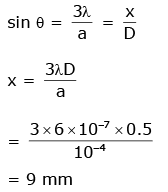JEE Main 2017 Question Paper with Solutions (9th April) - Question 24

A Laser light of wavelength 660 nm is used to weld Retina detachment. If a Laser pulse of width 60 ms and power 0.5 kW is used the approximate number of photons in the pulse are:
[Take Planck’s constant h=6.62×10−34 Js]

JEE Main 2017 Question Paper with Solutions (9th April) - Question 25

The acceleration of an electron in the first orbit of the hydrogen atom (n=1) is :

JEE Main 2017 Question Paper with Solutions (9th April) - Question 26

Imagine that a reactor converts all given mass into energy and that it operates at a power level of 109 watt. The mass of the fuel consumed per hour in the reactor will be: (velocity of light, c is 3×108 m/s)

JEE Main 2017 Question Paper with Solutions (9th April) - Question 27

The current gain of a common emitter amplifier is 69. If the emitter current is 7.0 mA, collector current is :

JEE Main 2017 Question Paper with Solutions (9th April) - Question 28

A signal is to be transmitted through a wave of wavelength λ, using a linear antenna. The length l of the antenna and effective power radiated Peff will be given respectively as:
(K is a constant of proportionality)

JEE Main 2017 Question Paper with Solutions (9th April) - Question 29

In a meter bridge experiment resistances are connected as shown in the figure. Initially resistance P=4 Ω and the neutral point N is at 60 cm from A. Now an unknown resistance R is connected in series to P and the new position of the neutral point is at 80 cm from A. The value of unknown resistance R is :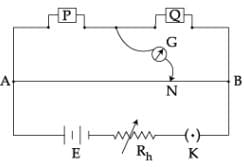JEE Main 2017 Question Paper with Solutions (9th April) - Question 30

In an experiment to determine the period of a simple pendulum of length 1 m, it is attached to different spherical bobs of radii r1 and r2. The two spherical bobs have uniform mass distribution. If the relative difference in the periods, is found to be 5×10−4 s, the difference in radii, |r1−r2| is best given by:

JEE Main 2017 Question Paper with Solutions (9th April) - Question 31

An ideal gas undergoes isothermal expansion at constant pressure. During the process :

JEE Main 2017 Question Paper with Solutions (9th April) - Question 32

50 mL of 0.2 M ammonia solution is treated with 25 mL of 0.2 M HCl. If pKb of ammonia solution is 4.75, the pH of the mixture will be:

JEE Main 2017 Question Paper with Solutions (9th April) - Question 33

The electron in the hydrogen atom undergoes transition from higher orbitals to orbital of radius 211.6 pm. This transition is associated with :

JEE Main 2017 Question Paper with Solutions (9th April) - Question 34

At 300 K, the density of a certain gaseous molecule at 2 bar is double to that of dinitrogen (N2) at 4 bar.  The molar mass of gaseous molecule is :

JEE Main 2017 Question Paper with Solutions (9th April) - Question 35

What quantity (in mL) of a 45% acid solution of a mono-protic strong acid must be mixed with a 20% solution of the same acid to produce 800 mL of a 29.875% acid solution?

JEE Main 2017 Question Paper with Solutions (9th April) - Question 36

To find the standard potential of M3+/M electrode, the following cell is constituted : Pt/M/M3+(0.001 mol L−1)/Ag+(0.01 mol L−1)/Ag The emf of the cell is found to be 0.421 volt at 298 K.  The standard potential of half reaction M3++3e→ M at 298 K will be :
(Given E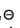Ag+/Ag at 298 K = 0.80 Volt)

JEE Main 2017 Question Paper with Solutions (9th April) - Question 37

A gas undergoes change from state A to state B. In this process, the heat absorbed and work done by the gas is 5 J and 8 J, respectively. Now gas is brought back to A by another process during which 3 J of heat is evolved. In this reverse process of B to A:

JEE Main 2017 Question Paper with Solutions (9th April) - Question 38

Adsorption of a gas on a surface follows Freundlich adsorption isotherm. Plot of log x/m versus log p gives a straight linewith slope equal to 0.5, then:

JEE Main 2017 Question Paper with Solutions (9th April) - Question 39

The rate of a reaction quadruples when the temperature changes from 300 to 310 K. The activation energy of this reaction is: (Assume activation energy and preexponential factor are independent of temperature; ln 2=0.693; R=8.314 J mol−1 K−1)

JEE Main 2017 Question Paper with Solutions (9th April) - Question 40

A solution is prepared by mixing 8.5 g of CH2Cl2 and 11.95 g of CHCl3. If vapour pressure of CH2Cl2 and CHCl3 at 298 K are 415 and 200 mmHg respectively, the mole fraction of CHCl3 in vapour form is: (Molar mass of Cl=35.5 g mol−1)

JEE Main 2017 Question Paper with Solutions (9th April) - Question 41

The electronic configuration with the highest ionization enthalpy is:

JEE Main 2017 Question Paper with Solutions (9th April) - Question 42

The following reaction occurs in the Blast Furnace where iron ore is reduced to iron metal:
Fe2O3(s)+3 CO(g) ⇌ 2 Fe(l)+3 CO2(g)
Using the Le Chatelier’s principle, predict which one of the following will not disturb the equilibrium?

JEE Main 2017 Question Paper with Solutions (9th April) - Question 43

Which one of the following is an oxide?

JEE Main 2017 Question Paper with Solutions (9th April) - Question 44

Which of the following is a set of green house gases?

JEE Main 2017 Question Paper with Solutions (9th April) - Question 45

The group having triangular planar structures is:

JEE Main 2017 Question Paper with Solutions (9th April) - Question 46

XeF6 on partial hydrolysis with water produces a compound ‘X’. The same compound ‘X’ is formed when XeF6 reacts with silica. The compound ‘X’ is :

JEE Main 2017 Question Paper with Solutions (9th April) - Question 47

The number of P −OH bonds and the oxidation state of phosphorus atom in pyrophosphoric acid (H4P2O7) respectively are :

JEE Main 2017 Question Paper with Solutions (9th April) - Question 48

Which of the following ions does not liberate hydrogen gas on reaction with dilute acids?

JEE Main 2017 Question Paper with Solutions (9th April) - Question 49

The correct sequence of decreasing number of π-bonds in the structures of H2SO3, H2SO4 and H2S2O7 is :

JEE Main 2017 Question Paper with Solutions (9th April) - Question 50

[Co2(CO)8] displays :

JEE Main 2017 Question Paper with Solutions (9th April) - Question 51

A compound of molecular formula C8H8O2 reacts with acetophenone to form a single cross-aldol product in the presence of base. The same compound on reaction with conc. NaOH forms benzyl alcohol as one of the products. The structure of the compound is :

JEE Main 2017 Question Paper with Solutions (9th April) - Question 52

Which of the following compounds is most reactive to an aqueous solution of sodium carbonate?

JEE Main 2017 Question Paper with Solutions (9th April) - Question 53

In the following structure, the double bonds are marked as I, II, III and IV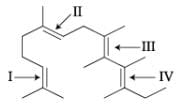Geometrical isomerism is not possible at site (s) :

JEE Main 2017 Question Paper with Solutions (9th April) - Question 54

The major product of the following reaction is: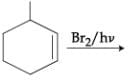JEE Main 2017 Question Paper with Solutions (9th April) - Question 55

The incorrect statement among the following is:

JEE Main 2017 Question Paper with Solutions (9th April) - Question 56

Which of the following is a biodegradable polymer?

JEE Main 2017 Question Paper with Solutions (9th April) - Question 57

The increasing order of the boiling points for the following compounds is :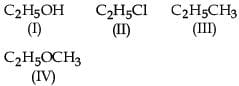JEE Main 2017 Question Paper with Solutions (9th April) - Question 58

Which of the following compounds will show highest dipole moment?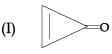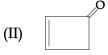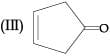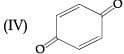JEE Main 2017 Question Paper with Solutions (9th April) - Question 59

In the following reaction sequence :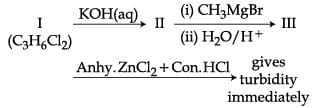The compound I is :

JEE Main 2017 Question Paper with Solutions (9th April) - Question 60

Among the following compounds, the increasing order of their basic strength is :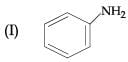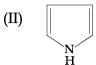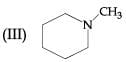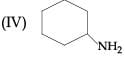JEE Main 2017 Question Paper with Solutions (9th April) - Question 61

The function f : N → N defined by f(x) = x-5 [x/5], where N is the set of natural numbers and [x] denotes the greatest integer less than or equal to x, is :

JEE Main 2017 Question Paper with Solutions (9th April) - Question 62

The sum of all the real values of x satisfying the equation 2(x−1)(x2+5x−50)=1 is :

JEE Main 2017 Question Paper with Solutions (9th April) - Question 63

The equation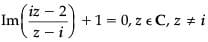represents a part of a circle having radius equal to :

JEE Main 2017 Question Paper with Solutions (9th April) - Question 64

For two 3 × 3 matrices A and B, let A+B=2B' and 3A+2B=I3, where B' is the transpose of B and I3 is 3×3 identity matrix. Then :

JEE Main 2017 Question Paper with Solutions (9th April) - Question 65

If x=a, y= b, z =c is a solution of the system of linear equations
x+8y+7z=0
9x+2y+3z=0
x+y+z=0
such that the point (a, b, c) lies on the plane x+2y+z=6, then 2a+b+c equals:

JEE Main 2017 Question Paper with Solutions (9th April) - Question 66

The number of ways in which 5 boys and 3 girls can be seated on a round table if a particular boy B1 and a particular girl G1 never sit adjacent to each other, is:

JEE Main 2017 Question Paper with Solutions (9th April) - Question 67

The coefficient of x−5 in the binomial expansion of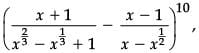where x ≠ 0, 1, is :

JEE Main 2017 Question Paper with Solutions (9th April) - Question 68

If three positive numbers a, b and c are in A.P. such that abc=8, then the minimum possible value of b is :

JEE Main 2017 Question Paper with Solutions (9th April) - Question 69

Let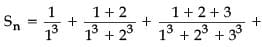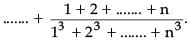If 100 Sn=n, then n is equal to:

JEE Main 2017 Question Paper with Solutions (9th April) - Question 70

The value of k for which the function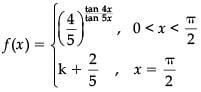is continuous at x = π/2, is:

JEE Main 2017 Question Paper with Solutions (9th April) - Question 71

If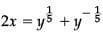and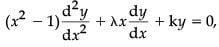then λ+k is equal to:

JEE Main 2017 Question Paper with Solutions (9th April) - Question 72

The function f defined by f(x)=x3−3x2+5x+7, is :

JEE Main 2017 Question Paper with Solutions (9th April) - Question 73

Let f be a polynomial function such that f (3x)=f'(x) ⋅ f''(x), for all x ∈ R.  Then :

JEE Main 2017 Question Paper with Solutions (9th April) - Question 74

If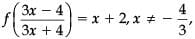and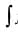f (x) dx = A log |1 − x| + Bx + C, then the ordered pair (A, B) is equal to :
(where C is a constant of integration)

JEE Main 2017 Question Paper with Solutions (9th April) - Question 75

If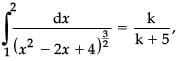then k is equal to:

JEE Main 2017 Question Paper with Solutions (9th April) - Question 76

If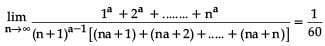for some positive real number a, then a is equal to :

JEE Main 2017 Question Paper with Solutions (9th April) - Question 77

A tangent to the curve, y= f(x) at P(x, y) meets x-axis at A and y-axis at B.  If AP : BP=1 : 3 and f(1)=1, then the curve also passes through the point :

JEE Main 2017 Question Paper with Solutions (9th April) - Question 78

A square, of each side 2, lies above the x-axis and has one vertex at the origin. If one of the sides passing through the origin makes an angle 30o with the positive direction of the x-axis, then the sum of the x-coordinates of the vertices of the square is :

JEE Main 2017 Question Paper with Solutions (9th April) - Question 79

A line drawn through the point P(4, 7) cuts the circle x2+y2=9 at the points A and B. Then PA⋅PB is equal to :

JEE Main 2017 Question Paper with Solutions (9th April) - Question 80

The eccentricity of an ellipse having centre at the origin, axes along the co-ordinate axes and passing through the points (4, −1) and (−2, 2) is:

JEE Main 2017 Question Paper with Solutions (9th April) - Question 81

If y=mx+c is the normal at a point on the parabola y2=8x whose focal distance is 8 units, then |c| is equal to:

JEE Main 2017 Question Paper with Solutions (9th April) - Question 82

If a variable plane, at a distance of 3 units from the origin, intersects the coordinate axes at A, B and C, then the locus of the centroid of ∆ABC is :

JEE Main 2017 Question Paper with Solutions (9th April) - Question 83

If the line,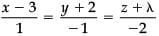lies in the plane, 2x−4y+3z=2, then the shortest distance between this line and the line,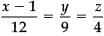is :

JEE Main 2017 Question Paper with Solutions (9th April) - Question 84

If the vector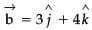is written as the sum of a vector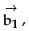parallel to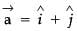and a vector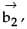perpendicular to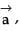then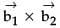is equal to:

JEE Main 2017 Question Paper with Solutions (9th April) - Question 85

From a group of 10 men and 5 women, four member committees are to be formed each of which must contain at least one woman. Then the probability for these committees to have more women than men, is:

JEE Main 2017 Question Paper with Solutions (9th April) - Question 86

Let E and F be two independent events. The probability that both E and F happen is 1/12 and the probability that neither E nor F happens is 1/2, then a value of P(E) / P(F) is :

JEE Main 2017 Question Paper with Solutions (9th April) - Question 87

The sum of 100 observations and the sum of their squares are 400 and 2475, respectively. Later on, three observations, 3, 4 and 5, were found to be incorrect. If the incorrect observations are omitted, then the variance of the remaining observations is :

JEE Main 2017 Question Paper with Solutions (9th April) - Question 88

A value of x satisfying the equation sin[cot−1(1+x)]=cos[tan−1x], is :

JEE Main 2017 Question Paper with Solutions (9th April) - Question 89

The two adjacent sides of a cyclic quadrilateral are 2 and 5 and the angle between them is 60o.  If the area of the quadrilateral is 4√3 , then the perimeter of the quadrilateral is:

JEE Main 2017 Question Paper with Solutions (9th April) - Question 90

Contrapositive of the statement
‘If two numbers are not equal, then their squares are not equal’, is:

## JEE Main & Advanced Mock Test Series

2 videos|325 docs|189 tests
 Use Code STAYHOME200 and get INR 200 additional OFF Use Coupon Code
Information about JEE Main 2017 Question Paper with Solutions (9th April) Page
In this test you can find the Exam questions for JEE Main 2017 Question Paper with Solutions (9th April) solved & explained in the simplest way possible. Besides giving Questions and answers for JEE Main 2017 Question Paper with Solutions (9th April), EduRev gives you an ample number of Online tests for practice

## JEE Main & Advanced Mock Test Series

2 videos|325 docs|189 tests